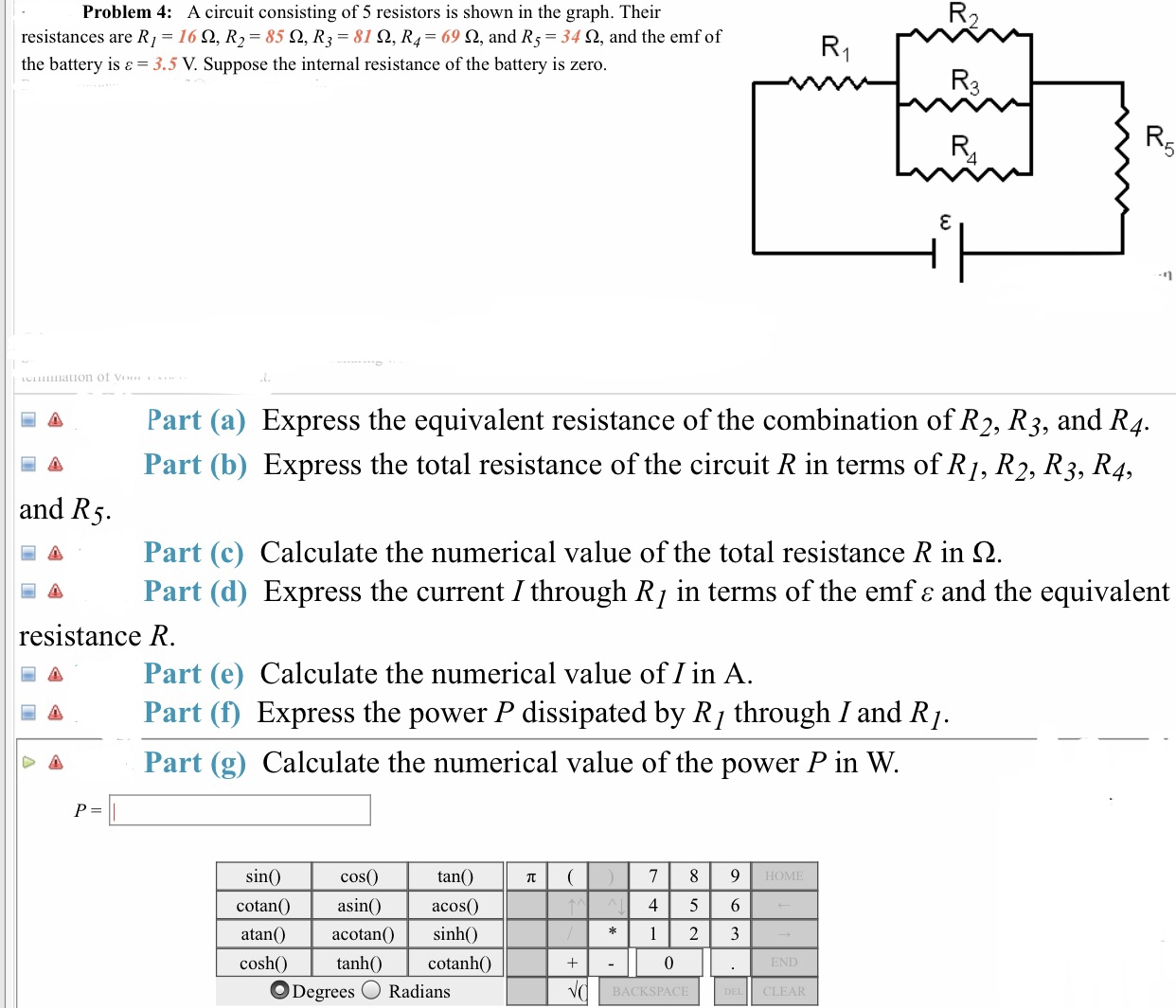# Problem 4: A circuit consisting of 5 resistors is shown in the graph. Theirresistances are R,-16 Ω, R,-85 Ω, R,-810, R4-69 Ω, and R5-34 Ω, and the emf ofthe battery is 3.5 V. Suppose the internal resistance of the battery is zeroPart (a) Express the equivalent resistance of the combination of R2, R3, and R4.Part (b) Express the total resistance of the circuit R in terms of R/, R2, R3, R4.and RPart(c) Calculate the numerical value of the total resistance R in ΩPart (d) Express the current I through Rj in terms of the emf e and the equivalentresistance RPart (e) Calculate the numerical value of I in Aa Part (f) Express the power P dissipated by R/ through I and R)Part (g) Calculate the numerical value of the power P in W.cos(0tan()sinHOMEcotanO asin acos) sinh04 5 6atan()acotan(coshcotanhOO Degrees RadiansBACKSPACECLEAR

Question
143 viewshelp_outlineImage TranscriptioncloseProblem 4: A circuit consisting of 5 resistors is shown in the graph. Their resistances are R,-16 Ω, R,-85 Ω, R,-810, R4-69 Ω, and R5-34 Ω, and the emf of the battery is 3.5 V. Suppose the internal resistance of the battery is zero Part (a) Express the equivalent resistance of the combination of R2, R3, and R4. Part (b) Express the total resistance of the circuit R in terms of R/, R2, R3, R4. and R Part(c) Calculate the numerical value of the total resistance R in Ω Part (d) Express the current I through Rj in terms of the emf e and the equivalent resistance R Part (e) Calculate the numerical value of I in A a Part (f) Express the power P dissipated by R/ through I and R) Part (g) Calculate the numerical value of the power P in W. cos(0 tan() sin HOME cotanO asin acos ) sinh0 4 5 6 atan()acotan( cosh cotanhO O Degrees Radians BACKSPACE CLEAR fullscreen
check_circle

### Want to see the full answer?

See Solution

#### Want to see this answer and more?

Solutions are written by subject experts who are available 24/7. Questions are typically answered within 1 hour.*

See Solution
*Response times may vary by subject and question.
Tagged in

### Physics